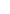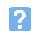1. In the transmitter block diagram shown below, which block represents the modulated RF amplifier stage?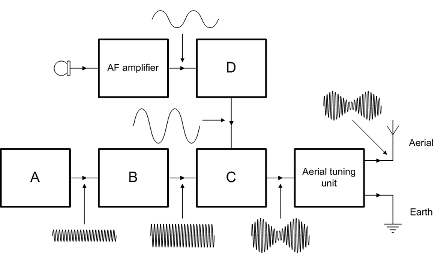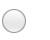a) Ab) Bc) Cd) D.2. In the arrangement shown, the function of the aerial tuning unit is to:a) act as a load for the output stageb) reduce the output power to a safe valuec) provide additional power gain before the aeriald) match the aerial to the output of the transmitter.3. In the radio receiver shown, the demodulator stage is the block marked: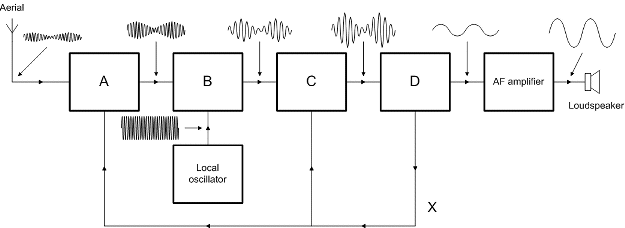a) Ab) Bc) Cd) D.4. In the radio receiver shown, the signal marked X is:a) front-end tuningb) automatic gain controlc) automatic frequency controld) positive feedback to improve stability.5. In the circuit shown, the signal appearing at the input and output of the block marked C will typically be:a) 10.7 kHzb) 10.7 MHzc) in the range 88 MHz to 108 MHzd) in the range 455 kHz to 470 kHz.6. In the circuit shown, the majority of gain and selectivity is achieved in the block marked:a) Ab) Bc) Cd) D.7. In the diagram shown, which of the curves represents critical coupling between two tuned circuits?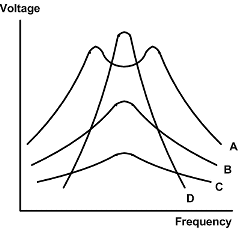a) Ab) Bc) Cd) D.8. In the demodulator circuit shown, R1 and C2 form: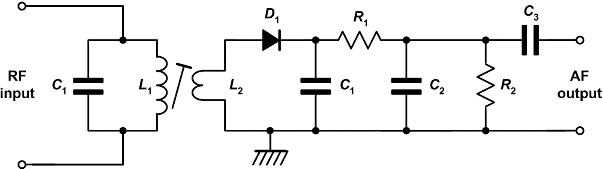a) a C-R time delay circuit that slows the IF signalb) a low-pass filter that removes the residual IF signalc) a high-pass filter that accepts the RF and IF signalsd) a C-R circuit that reduces the amplitude of all incoming signals.9. The dimension marked L in the diagram below is typically: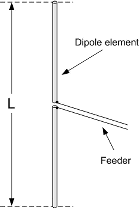a) a half of the wavelength of the signal to be receivedb) an eighth of the wavelength of the signal to be receivedc) a quarter of the wavelength of the signal to be receivedd) three quarters of a wavelength of the signal to be received.10. The aerial shown in the diagram below is: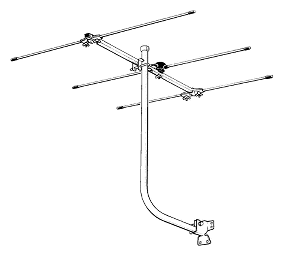a) a vertically polarised directional aerialb) a horizontally polarised directional aerialc) a vertically polarised omnidirectional aeriald) a horizontally polarised omnidirectional aerial.
This is more feedback!
This is the feedback!